# Shattering of Arbitrary Computer Graphics Models

By Rob Engle and Andrea Rosso

Introduction
Limiting the Problem
Input
Subdivision of Objects
Physical Model
Our "Model"
Particle System
Collision Detection
Cracking Algorithms
Output
Results
More Animations!
Conclusions and Further Work

## Introduction

This project was done for CS 348C - Modeling in Computer Graphics. The initial aim of our project was to create realistic looking explosions using computer graphics modeling techniques. There are numerous reasons why one would want to simulate explosions. The most important one seems to be for motion picture visual effects. There are many instances in film making, such as in sci-fi or action films, where an object needs to be exploded. Even when most of the images of an object are computer modeled, most film makers seem to rely on traditional pyrotechnics and a physical model in order to achieve a realistic explosion. By implementing an algorithm to do it in computer graphics, a lot of work and time would be saved and, perhaps, more control over the final effect could be achieved. Our goal was to try and create an algorithm that would obtain reasonable results for a limited problem domain. What we propose in this project is by no means a complete solution, but it is a step in the right direction. Very little (computer graphics) literature was available on the subject so we tried to base our methods on heuristics.

## Limiting the Problem

There are at least four distinct sections to an explosions which need to be simulated to achieve a complete effect:
-The Fireball
-The Flaming Debris
-The Smoke
-The Shattering of the Object
These are all very complex problems in themselves. Since this was a quarter project we decided to focus on only one of these parts, mainly the problem of having an object submitted to an explosive force shatter in a realistic way. Our objective was to shatter an arbitrary object or objects given some forces acting upon it.

## Input

Our first concern was to read in the objects using some generally available modeling language. We chose to use
Virtual Reality Modeling Language (VRML) because of the availability of a free reader library. Also a large number of models existed for it. Our expectation was that we could convert SOFTIMAGE 3D scenes to VRML (via Open Inventor) without running into too many difficulties. Unfortunately it turned out that things like camera parameters were lost in the conversion.

## Subdivision of Objects

In order to make the shattering of the objects look realistic we had to subdivide each object into smaller patches or polygons. We chose to use triangles which have some nice properties (e.g.: they are guaranteed to be planar and all VRML objects can be subdivided into them) about them, and are easy to deal with. Our algorithm would subdivide the object and keep track of how the triangles were interconnected. This meant that at each triangle we had a data structure containing information about the adjacent triangles.

## Physical Model

Our first intuition was to model the shattering of the object by using a direct physical model where we would calculate the forces acting upon all the triangles. We soon realized that this is a very expensive process, since the force on every triangle is also dependent on the force of every other triangle connected to it. Solving such a set of equations is very expensive and time grows as the cube of the number of triangles. So we quickly realized this was not the way to go.

## Our "Model"

Our model consisted of three different parts: A particle system, a collision detection system, and some cracking algorithms. Here is what each of these does:

### Particle System

We implemented a general particle system, where each particle would keep track of its position and its rotational and translational velocities. We used this particle system in order to move our shattered pieces around the scene. What we would do is associate a particle with each connected group of triangles and update them accordingly each time the particle was updated. Each particle would move according to forces in the scene and follow the F=ma equation. We could specify different types of forces for a particular scene: Radial forces, Gravity, Constant forces, Random forces, and any scale or combination of these.

### Collision Detection

We also wanted the shattering objects in our scene to interact with each other. For instance, if we put a floor beneath the object or walls around the object, we would have liked the different pieces to bounce off as expected. This turned out to be more complicated than expected and we did not have time to implement a complete solution.

### Cracking Algorithms

We initially cracked the surface of the objects by simply disconnecting all the triangles and letting them fly off according to the force exerted upon them. This looked decent but it was not enough to make the shattering look realistic. One common characteristic of explosions is that the individual pieces of flying debris have a wide variance of shape and size. So we decided to implement different algorithms that would traverse the surface and break it apart according to some method. All our methods were based on probability. In each, the probability of breaking a link was weighted by some heuristic that would determine how the surface would crack. Here are the algorithms we implemented:
Completely Random: Here we did not weight the probabilities and let the cracking be completely random.
Path of Minimum Force: Here we weighted the probabilities so that it was more likely that we wouldn't break links that had less force exerted on them.
Path of Minimum Normal difference: Here we weighted the probabilities by the difference of the current normal and the next normal. In this way we would try to pick the path that would keep us in the same general area.
Path of Minimum Distance: Here we weighted the probabilities by using the geometric distance between the triangles' centers of mass. This also would keep us in the same general area in order to keep "chunky" pieces.

## Output

For the output of our program we used
RenderMan Interface Bytestream (RIB) files. This enabled us to use Larry Gritz's Blue Moon Rendering Tools (BMRT) or Pixar's PhotoRealistic RenderMan to render our scenes. Also, it gave us a very flexible system with which to render our scene. For this project we made and modified a couple of RenderMan shaders to produce glass and other materials. We did not have time to use them to their full potential because of time constraints.

In our animation we had a few extra features that are worth mentioning. We first of all had the option of rendering thick primitives. Generally objects were described as a surface, with no concept of thickness. This is enough for solid objects, but once the object breaks apart we have to make it look like the object had thickness in the first place. What was done here is that we would extrude each triangle along its normal and create 4 more primitives (the back and sides of each triangle) in order to make each piece look thick. Another feature we added was motion blur. Since objects in our scene had a tendency to move very quickly because of the extreme forces, we needed to make the transition between frames as smooth as possible. We did this by keeping track of how the object moved throughout the sequence and giving RenderMan the appropriate information with which to blur.

## Results

We created four animations to show the effect of the different cracking algorithms. Each one shows a sphere being affected by a radial force offset a little to the right of the sphere center. The animations are: Completely Random algorithm, Path of minimum force, Path of minimum normal difference, Path of minimum distance. Here are also four stills showing each results: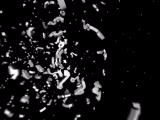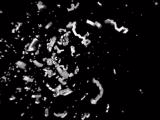Completely Random ------- Minimum Force Path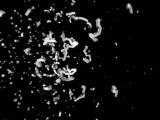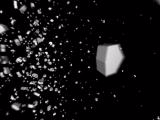Minimum Distance Path ------- Minimum Normal Diff.

You'll notice that the path of minimum normal difference looks the best, and has the desired "chunky" effect to it. In the random force we get a completely random break up which does not look too good. For the path of minimum force you'll notice that the pieces are long strings of triangles which flow away from the explosions epicenter. Finally, the minimum distance criteria did not work very well because it took into account the geometrical distance and not the surface distance and because our algorithm was completely local (once a decision to link two triangles was made, we never went back to the original triangle... we tended to move away from the original triangle).

## More animations!

Here are a couple more animations:
Teapot and World. The teapot animation shows how we could explode an arbitrary object, and the world animation shows how we kept track of texture coordinates.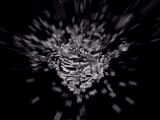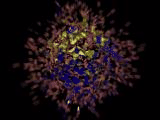## Conclusions and Further Work

There are many things we could not complete by the time of the presentation. Collision detection wasn't fully functional and this limited the types of animations we could do. Also, we wasted a lot of time trying to get all the conversions from the different formats into our program and out for rendering. The main problem seems to stem from the fact that different implementations use different standards for defining their surface normals and this caused a lot of problems.

Finally, we have a few ideas for future work which we didn't have time to implement. The first is for the cracking algorithms. If we were to subdivide triangles more depending on the force exerted upon them, we believe we would get a more realistic effect, since objects tend to shatter more at points of extreme force. We could also try to come up with a more physically based model, by trying to only take into account interaction of forces between neighboring triangles, instead of all the triangles in the object. This would lead to much more solvable system of equations. Finally we could extend our models to have areas of strength, which determine how strong a certain area of an object is when a force is exerted on it. This would enable us to simulate the breaking of edges, for instance, more realisticly.

For further information please mail us:
Rob Engle and Andrea Rosso